# Solution of Right Angled Triangle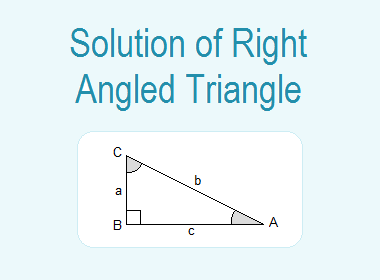## Solution of Right Angled Triangle

A triangle has three sides and three angles. Angles and sides together are said to be the six parts of a triangle.

********************

10 Math Problems officially announces the release of Quick Math Solver, an Android App on the Google Play Store for students around the world.

********************

In a right-angled triangle, one angle is 90°, so there are 5 parts unknown.

Under a given condition, the finding of the unknown parts of a right angled triangle is known as the solution of a right-angled triangle.Let ABC be a right-angled triangle, right-angled at B. We denote its angles CAB, ABC, and BCA by A, B, and C respectively.Here, B = 90°.

The sides opposite to angles A, B, and C are denoted by a, b and c respectively. So, BC = a, CA = b and AB = c.

Then,

By Pythagoras relation h2 = p2 + b2, we have

b2 = a2 + c2

Again, by the sum of two acute angles,

A + C = 90°

If we know one side and any one of the remaining four parts, we can find the other parts of the right-angled triangle. This process of calculating the unknown parts is known as the solution of a right-angled triangle.

### Methods of Solving a Right Angled Triangle

#### - When two sides of a right-angled triangle are given

(i)        When a and c, are given.

Then, b2 = a2 + c2.

b = √(a2 + c2)

Again, sinA = a/b and so A is found.

At last, C = 90° - A.

(ii)     When, a and b are given.

Then, c2 = b2 – a2.

c = √(b2 – a2)

Again, sinA = a/b and it gives A.

At last, C = 90° - A.

#### - When one side and one acute angle are given

(i)        When A and b are given.

Then, C = 90° - A

sinA = a/b

a = b sinA

cosA = c/b

c = b cosA

(ii)     When, A and a are given.

Then, C = 90° - A

sinA = a/b

b = a/sinA = a cosecA

cosA = c/b

c = b cosA

### Worked Out Examples

Example 1: Find the unknown angles and the sides of the following right angled triangles:

Solution:

(i)        Figure: Right angled triangle ABCIn DABC, B = 90°, C = 45° and BC = √2

A = 90° – C = 90° – 45° = 45°

Now,

tan45° = AB/BC

or,   1 = AB/√2

or,   AB = √2

Again,

cos45° = BC/AC

or,   1/√2 = √2/AC

or,   AC = 2

A = 45°, AB = √2 and AC = 2  Ans.

(ii)     Figure: Triangle ABC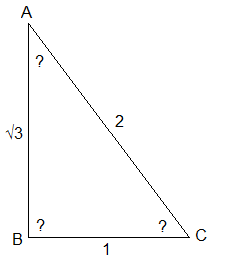In triangle ABC,  AB = √3, BC = 1 and AC = 2

Now,

AB2 + BC2 = (√3)2 + (1)2

= 3 + 1

= 4

= (2)2

= AC2

Since, AB2 + BC2 = AC2

B = 90°

Now,

sinC = AB/AC

or,   sinC = √3/2

or,   sinC = sin60°

C = 60°

And, A = 90° – C = 90° – 60° = 30°

B = 90°, C = 60°, and A = 30° Ans.

Example 2: Solve the right angled triangle ABC in which C = 90°, B = 30° and a = 4√3 cms.Solution: Here,

In DABC, C = 90°, B = 30° and a = BC = 4√3 cms.

A = 90° – C = 90° – 30° = 60°

Now,

cos30° = BC/AB

or,     √3/2 = 4√3/AB

or,     AB√3 = 8√3

or,     AB = 8√3/√3 = 8 cms.

Again,

sin30° = AC/AB

or,     1/2 = AC/8

or,     2AC = 8

or,     AC = 8/2 = 4 cms.

c = AB = 8cms, b = AC = 4cms, and A = 60°  Ans.

Example 3: In a right angled triangle ABC, if B = 90°, a = 2√3 and b = 4, find the remaining angles and the sides.Solution: Here,

In triangle ABC,

B = 90°

a = BC = 2√3

b = AC = 4

Now,

cosC = BC/AC = 2√3/4 = √3/2 = cos30°

C = 30°

And,

A = 90° – C = 90° – 30° = 60°

Again,

sin30° = AB/AC

or,     1/2 = AB/4

or,     2AB = 4

or,     AB = 4/2 = 2

A = 60°, C = 30°, and c = AB = 2  Ans.

Example 4: Solve the triangle ABC if A = 90°, b = 16 and c = 16.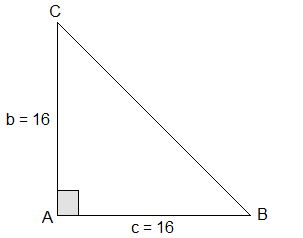Solution: Here,

In triangle ABC,

A = 90°

b = AC = 16

c = AB = 16

Since A = 90°, so a is the hypotenuse.

a2 = b2 + c2

= (16)2 + (16)2

= 2 × (16)2

or,  a = 16√2

Now,

tanB = AC/AB = b/c = 16/16 = 1 = tan45°

B = 45°

And,

C = 90° – B = 90° – 45° = 45°

B = 45°, C = 45° and a = BC = 16√2 Ans.

Example 5: Solve the triangle ABC if a = 5√3, b = 5 and c = 10.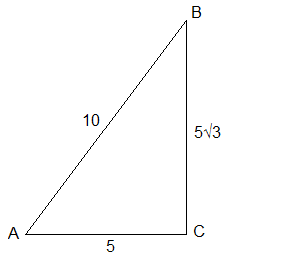Solution: Here,

In triangle ABC,

a = BC = 5√3

b = AC = 5

c = AB = 10

Now,

AC2 + BC2 = (5)2 + (5√3)2

= 25 + 75

= 100 = (10)2 = AB2

Since,  AC2 + BC2 = AB2

C = 90°

Now,

cosA = AC/AB = 5/10 = ½ = cos60°

A = 60°

And,

B = 90° – A = 90° – 60° = 30°

C = 90°, B = 30°, and A = 60° Ans.

Example 6: Solve a triangle ABC if B = 60°, A = 30° and a = 20.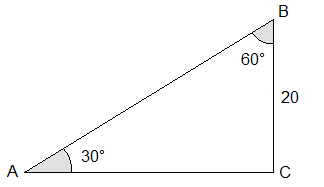Solution: Here,

In triangle ABC,

B = 60°

A = 30°

a = BC = 20

Now,

C = 180° – (A + B)

= 180° – (30° + 60°)

= 180° – 90°

= 90°

And,

sin30° = BC/AB

or,     1/2 = 20/AB

or,     AB = 40

Again,

cos30° = AC/AB

or,     √3/2 = AC/40

or,     2AC = 40√3

or,     AC = 40√3/2 = 20√3

C = 90°, c = AB = 40 and b = AC = 20√3 Ans.

Example 7: In a right angled triangle the sides containing the right angle are 4√3cm and 12cm. Find the hypotenuse and acute angles.Solution: Here,

In triangle ABC,

B = 90°

c = AB = 4√3cm.

a = BC = 12cm.

Now,

tanC = AB/BC = 4√3/12 = 1/√3 = tan30°

C = 30°

And,

A = 90° – C = 90° – 30° = 60°

Again,

sinC = AB/AC

or,     sin30° = 4√3/AC

or,     ½ = 4√3/AC

or,     AC = 8√3 cm.

A = 60°, C = 30°, and hypotenuse = AC = 8√3cm. Ans.

Example 8: Solve the triangle ABC if a = 3, b = 4 and c = 5 [Take cos54° = 3/5]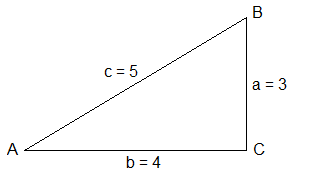Solution: Here,

In triangle ABC,

a = BC = 3

b = AC = 4

c = AB = 5

Now,

a2 + b2 = 32 + 42 = 9 + 16 = 25 = 52 = c2

i.e.  a2 + b2 = c2

C = 90°

Again,

cosB = a/c = 3/5 = cos54°

B = 54°

And,   A = 90° – B

= 90° – 54°

= 36°

A = 36°, B = 54° and C = 90° Ans.

If you have any questions or problems regarding the Solution of Right Angled Triangle, you can ask here, in the comment section below.